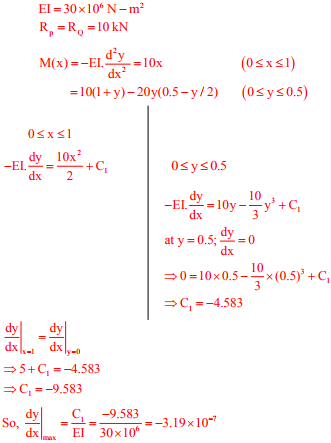# Structural Engineering - Online Test

Q1. A uniformly distributed line load of 500 kN/m is acting on the ground surface. Based on Boussinesq’s theory, the ratio of vertical stress at a depth 2 m to that at 4 m, right below the line of loading, is
Explaination / Solution:

Due to UDL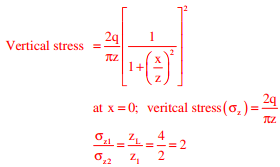Q2. According to IS 456-2000, which one of the following statements about the depth of neutral axis Xu,bal for a balanced reinforced concrete section is correct?
Explaination / Solution:

By limit state method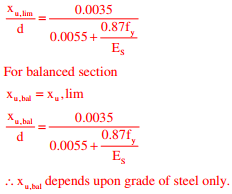Q3. The figure shows a two-hinged parabolic arch of span L subjected to a uniformly distributed load of intensity q per unit length.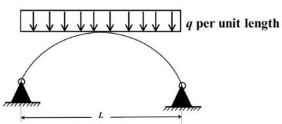The maximum bending moment in the arch is equal to
Explaination / Solution:

Bending moment at any point for two-hinged parabolic arch with uniformly distributed load is zero.

Q4. An elastic bar of length L, uniform cross sectional area A, coefficient of thermal expansion α, and Young’s modulus E is fixed at the two ends. The temperature of the bar is increased by T, resulting in an axial stress σ. Keeping all other parameters unchanged, if the length of the bar is doubled, the axial stress would be
Explaination / Solution: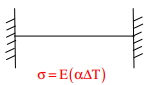σ varies with ∆T and does not depends upon the length of bar.

Q5. A strip footing is resting on the ground surface of a pure clay bed having an undrained cohesion Cu. The ultimate bearing capacity of the footing is equal to
Explaination / Solution:

Ultimate bearing capacity = c.Nc
For pure clay N= 5.14 = (π + 2)
UBC = (π + 2)C

Q6. A simply supported beam is subjected to a uniformly distributed load. Which one of the following statements is true?
Explaination / Solution: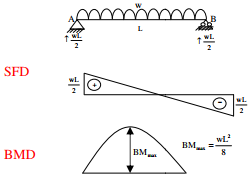Q7. A planar truss tower structure is shown in the figure.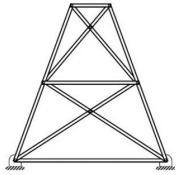Consider the following statements about the external and internal determinacies of the truss.
(P) Externally Determinate
(Q) External Static Indeterminacy = 1
(R) External Static Indeterminacy = 2
(S) Internally Determinate
(T) Internal Static Indeterminacy = 1
(U) Internal Static Indeterminacy = 2
Which one of the following options is correct?
Explaination / Solution: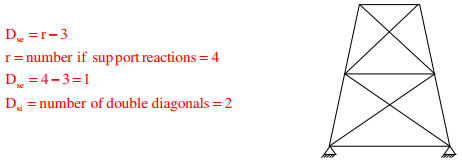Q8. The following observations are made while testing aggregate for its suitability in pavement construction:
i. Mass of oven-dry aggregate in air = 1000 g
ii. Mass of saturated surface-dry aggregate in air = 1025 g
iii. Mass of saturated surface-dry aggregate under water = 625 g
Based on the above observations, the correct statement is
Explaination / Solution:

Mass of oven dry aggregate wa = 1000g
Mass of water in saturated surface dry aggregate = ww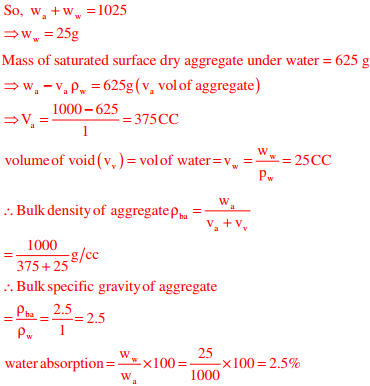Q9. Consider the plane truss with load P as shown in the figure. Let the horizontal and vertical reactions at the joint B be HB and reaction at the joint C.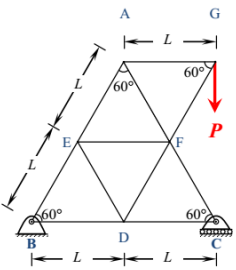Which one of the following sets gives the correct values of VBHB and VC?
Explaination / Solution: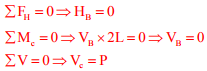Q10. A 3 m long simply supported beam of uniform cross section is subjected to a uniformly distributed load of w = 20 kN/m in the central 1 m as shown in the figure.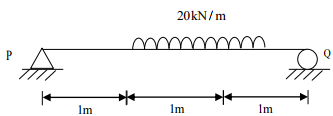in radians) of the deformed beam is
Explaination / Solution: